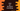# Java program to get the epoch timestamp## What is epoch time:

Epoch time is the number of seconds passed since 1st January 1970, 00:00 UTC. This time is used in many ways in software development. For example, if we are storing the modification time for a file in epoch, we can compare it with the current time and convert it to any timezone.

In almost all programming languages, there is a way to find the epoch time. In Java, we have the System class, that provides the method currentTimeMillis to calculate epoch time.

### Method 1: currentTimeMillis:

This is a static method defined in the System class as below:

``public static native long currentTimeMillis();``

It is the time difference in milliseconds between the current time and midnight, January 1, 1970 UTC.

We can convert this time to seconds by dividing the value by 1000.

### Example program:

Let’s take a look at the below program:

``````public class Example {
public static void main(String[] args) {
long currentTimeMillis = System.currentTimeMillis();
long currentTimeSeconds = currentTimeMillis/1000;

System.out.println("Current time in milliseconds: "+currentTimeMillis);
System.out.println("Current time in seconds: "+currentTimeSeconds);
}
}``````

It will print the current time in both milliseconds and seconds.

### Method 2: Using Instant class:

java.time.Instant is a useful class to record event timestamps in Java applications. This class provides one way to get the epoch time. use now() to get the current Instant use toEpochMilli() to get epoch time in milliseconds. It will be:

``````import java.time.Instant;

public class Example {
public static void main(String[] args) {
long currentTimeMillis = Instant.now().toEpochMilli();

System.out.println("Current time in milliseconds: "+currentTimeMillis);
}
}``````

### Method 3: By using the Date class:

Date class provides one method called getTime that returns the epoch time in milliseconds. It returns one long value.

``public long getTime()``

It looks as like below:

``````import java.util.Date;

public class Example {
public static void main(String[] args) {
long currentTimeMillis = new Date().getTime();

System.out.println("Current time in milliseconds: "+currentTimeMillis);
}
}``````

You can use any of these three methods we discussed in this post. All will give the same result.